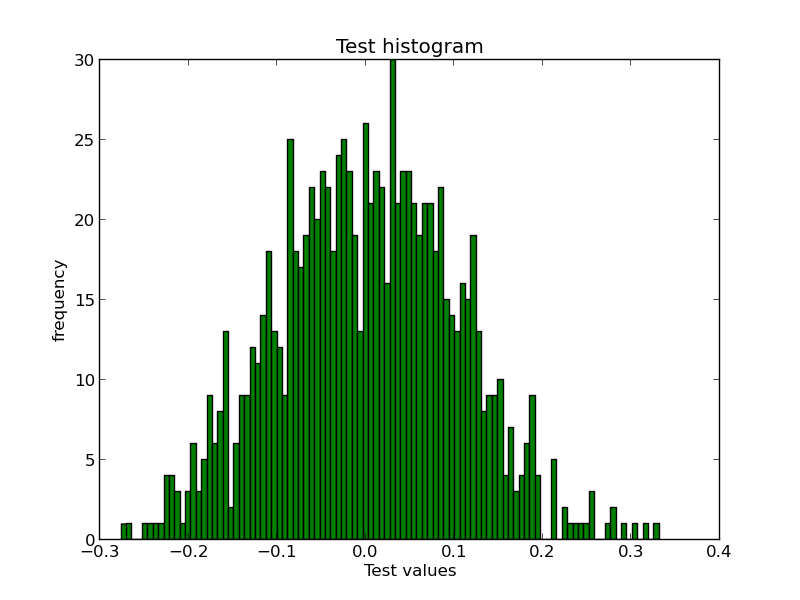# Toolbox: histit.py

(This is the first post of the series I plan to write on some tools I have built over the last couple of years to help me in my day-to-day tasks. I usually put all such tools in the repo toolbox until they turn out sufficiently useful to be worth separating into their own projects).

The first post is about a command line tool named `histit.py` that I use for quickly plotting histograms 1 by reading data from text files. It's basically a simple wrapper over matplotlib, a library that's quite popular for plotting all kinds of stuff in Python.

```    \$ wget https://raw.github.com/naiquevin/toolbox/master/histit.py
```

`hisit.py` depends upon numpy and matplotlib so first make sure they are installed 2

We need some data for the demo, so let's first dump values from a random normal distribution into a text file. The `numpy.random.normal` function can be used for this.

```    In : import numpy as np
In : with open('data.txt', 'w') as f:
...:     f.write('\n'.join(str(float(x)) for x in np.random.normal(0, 0.1, 1000)))
...:
```

Now we can use `histit.py` to plot the histogram as follows:

```    \$ python histit.py "Test histogram" "Test values" -d data.txt -a show -t float -b 100
```

If all goes well, a window 3 will popout with the histogram as shown below. Although the values in your case would be different, a peculiar "bell curve" should be noticeable.In the command we just run,

• "Test histogram" is the title of the plot,
• "Test values" is the label on the X-axis.
• `-d` option is for specifying the path to the data file
• `-a` option is for action (either of `show` and `save`, `show` being the default)
• `-t` option is for specifying the type of input expected, here `float` but the default is `int`
• `-b` option for specifying the no. of bins

Instead of loading data from a file, the script can also accept data on the standard input stream in which case, the `-d` option must be skipped.

```    \$ cat data.txt | python histit.py "Test histogram" "Test values" -a show -t float -b 100
```

I have been using this on a regular basis for some time but I am not entirely convinced if it's worth publishing as a package on PyPI. Anyway, at least it's got some documentation now :-)

#### Footnotes

1. A histogram is a graphical representation of frequency distribution. In simpler words, a graph of all unique values in the data plotted against how many times each one appears. When working with sufficient quantity of data, a histogram turns out to be a pretty handy tool to guage the shape of the data at a glance. See also: wikipedia

2. Actually it doesn't depend on numpy directly but before installing matplotlib make sure numpy is installed in order to avoid frustation.

3. GTK window in my case as I am using the GTKAgg backend for matplotlib. Please consult the docs to configure your preferred backend.

---